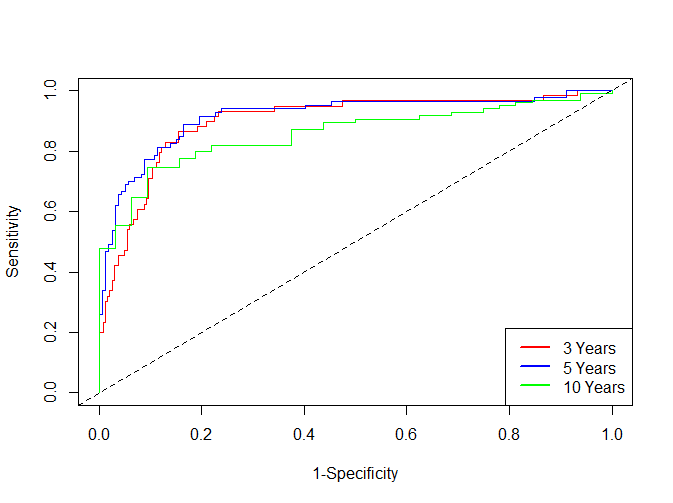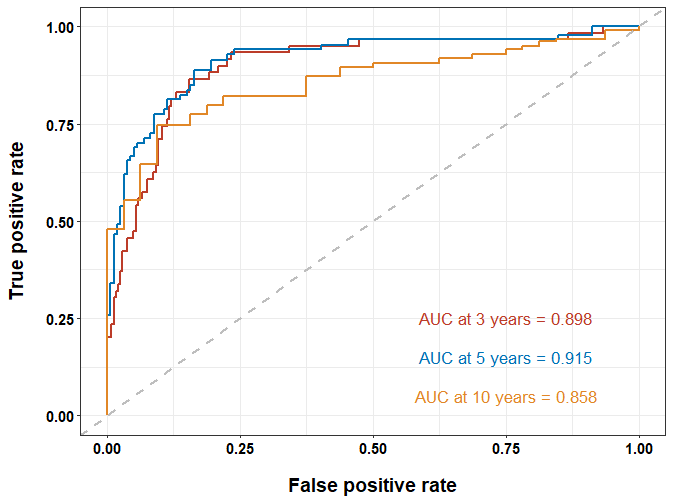Posted on

time-dependent ROC曲线的原理与常规的ROC曲线比较类似，前者相比后者多了时间因素，以便我们可以根据不同时间节点绘制不同的ROC曲线

timeROC包相比survivalROC包会多计算个AUC的置信区间

``````library(timeROC)
library(survival)

data(mayo)

time_roc_res <- timeROC(
T = mayo\$time,
delta = mayo\$censor,
marker = mayo\$mayoscore5,
cause = 1,
weighting="marginal",
times = c(3 * 365, 5 * 365, 10 * 365),
ROC = TRUE,
iid = TRUE
)
``````

``````> time_roc_res\$AUC
t=1095    t=1825    t=3650
0.8982790 0.9153621 0.8576153

> confint(time_roc_res, level = 0.95)\$CI_AUC
2.5% 97.5%
t=1095 85.01 94.64
t=1825 87.42 95.65
t=3650 79.38 92.14
``````

``````plot(time_roc_res, time=3 * 365, col = "red", title = FALSE)
plot(time_roc_res, time=5 * 365, add=TRUE, col="blue")
plot(time_roc_res, time=10 * 365, add=TRUE, col="green")
legend("bottomright",c("3 Years" ,"5 Years", "10 Years"),
col=c("red", "blue", "green"), lty=1, lwd=2)
``````time-

``````time_ROC_df <- data.frame(
TP_3year = time_roc_res\$TP[, 1],
FP_3year = time_roc_res\$FP[, 1],
TP_5year = time_roc_res\$TP[, 2],
FP_5year = time_roc_res\$FP[, 2],
TP_10year = time_roc_res\$TP[, 3],
FP_10year = time_roc_res\$FP[, 3]
)
library(ggplot2)
ggplot(data = time_ROC_df) +
geom_line(aes(x = FP_3year, y = TP_3year), size = 1, color = "#BC3C29FF") +
geom_line(aes(x = FP_5year, y = TP_5year), size = 1, color = "#0072B5FF") +
geom_line(aes(x = FP_10year, y = TP_10year), size = 1, color = "#E18727FF") +
geom_abline(slope = 1, intercept = 0, color = "grey", size = 1, linetype = 2) +
theme_bw() +
annotate("text",
x = 0.75, y = 0.25, size = 4.5,
label = paste0("AUC at 3 years = ", sprintf("%.3f", time_roc_res\$AUC[])), color = "#BC3C29FF"
) +
annotate("text",
x = 0.75, y = 0.15, size = 4.5,
label = paste0("AUC at 5 years = ", sprintf("%.3f", time_roc_res\$AUC[])), color = "#0072B5FF"
) +
annotate("text",
x = 0.75, y = 0.05, size = 4.5,
label = paste0("AUC at 10 years = ", sprintf("%.3f", time_roc_res\$AUC[])), color = "#E18727FF"
) +
labs(x = "False positive rate", y = "True positive rate") +
theme(
axis.text = element_text(face = "bold", size = 11, color = "black"),
axis.title.x = element_text(face = "bold", size = 14, color = "black", margin = margin(c(15, 0, 0, 0))),
axis.title.y = element_text(face = "bold", size = 14, color = "black", margin = margin(c(0, 15, 0, 0)))
)
``````## 10 Replies to “绘制Time-dependent ROC 曲线”

1.士绅说道：

你好，进哥哥，请问我按照一样的处理方法处理了3个数据集到了跑timeROC这一步，其中一个数据集提示Error in Mat_data_cont1[, c(“T”, “marker”)] :
incorrect number of dimensions，但是我的T和marker长度是一致的，也都是数值型，请问你知道这个问题该如何解决吗？

2.说道：

老师，您好，请问怎么求最佳cutoff呢？以及时间依赖ROC曲线可以联合多指标吗？

1.进哥哥说道：

这个ROC曲线用的是连续变量，不存在cutoff，用来评估生存数据的
R做生存分析如何取最佳cutoff(截断)
没明白联合多个指标什么意思？

3.LSH说道：

老师您好，我想问一下我画出来ROC曲线，一开始有一小部分在虚线下面，后面的部分又都在虚线的上面了，想问一下这样子画出来的ROC曲线是正确的吗

1.进哥哥说道：

您好，可能存在这样的情况，这样的数据出来的结果不太好

1.LSH说道：

您好，我是给验证集做ROC曲线的时候出现了这样子的情况，但是AUC值是0.7多，那出现这样子的情况是否代表训练集中构建的模型预后效果是不太好的呢

1.进哥哥说道：

0.7多一点，看你的要求吧，如果验证集也满意 大于0.7 那就还行
你应该画的是1年的ROC吧 可以看看3/5年的是多少

1.LSH说道：

好的，谢谢麻烦您啦

4.monica说道：

请问根据这个代码可以得出准确度和敏感性这些指标吗？

1.进哥哥说道：

什么意思？ROC曲线不就是翻译敏感性与特异性之间关系